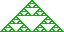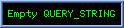Generalised 5D Dirac Particle

The essence of the Â1,3  Free Dirac-Hestenes equation represents ensuring Ñp2yp = -m2yp by insisting insisting that Ñpyp = imypg0 and associating i with right-multiplication by e12. It is approached this way because physicicsts want the Dirac-real momentum-operator mE(t) = hi Ñp to act like a 1-vector operator and so have grade 1.
We end up with Ñpyp = mypg012 exploiting (g012)2=-1 to solve Ñp2yp=-m2yp.

The direct Â3,1 equivalent is Ñp[e1234]yp = mypie124 for some i commuting with e124 and exploiting e1242=1  to solve Ñp2yp=-m2yp.    We might for example take i=e4 and consider a planewave solution   yp = ((m¿p)e12)y0' for any fixed y0' commuting with e124 , representing a "twist" in e12 "progressing" along m .

The obvious generalisation of this idealised approach is yp = ypa with Ñpyp = ypb where b2a=-m2a so that, provided a and b are p-independant, we have Ñp2yp = ypb2a = -m2yp .
Taking a=u=½(1±e124)½(1±e345) means that b2 can lie within the commuting algebra Algebra[e124,e345] as b2 = a1e124 +a2e345 +a3e135 - m2 - a1 - a2 - a3 and satisfy b2u = (b*b)u = -m2a . Further b2 may contain any left multiples of anything annihilated by u

Further Idempotents
We have associated idempotents ¼(1-e124)(1±e345) corresponding to matrices with 1 in the second and third lead diagnonal positions with the electrons of spin ±½e12 and ¼(1+e124)(1±e345) corresponding to 1 in the first and fourth with the positron.
Because these four idempotents annihilate eachother, we can construct a further six idempotents with scalar part ½ by adding any two of them, and a further four with scalar part 3/4 by adding any three. Adding all four gives 1 so, excluding unity, we have fifteen commuting (but not annihilating) Clifford-real (a§#=a) and e4-Hermitian-real (a=a) idempotents in the commutative algebra generated by e124 and e345 coresponding to C4×4 matrices with one, two, or three 1s on the lead diagonal and 0s elsewhere. ½(1±e124) correspond to charged nonspinning particles while ½(1±e345) correspond to uncharged particles with spin e12.
These further idempotents do not all satisy Â4,1 geometry-invariant condition uu§=0 nor do they all absorb e124,e345, and e1234 with sign changes. They do however all have an associated unit plussquare 3-blade b=½(1-u) with ub=-u When not annihilating eachother, the different u "absorb" with for example (u+u')u=u.

Simple Solutions
Of course, any idempotemt u provides unit plussquare b=1-2u with ub = ub = -u and we have
yp = rp m ((m¿p+qp)ib) u satisfying Ñp yp = ((Ñprp)rp-1 - im - i(Ñpqp))yp provided i has i2=-1 and commutes with u and m. We will favour i=i=e12345 here.
If rp=r, and qp=q we have Ñpyp = imyp which vanishes for null five-momentum , ie. m5 = positive rest mass for timelike four-momentum.
[ Proof : Ñp yp   =   rp m2 ((m¿p+qp)ib) bu + (Ñprp)rp-1 yp + (Ñpqp)rp m((m¿p+qp)ib) bu   =   ((Ñprp)rp-1 - mi - (Ñpqp)i)yp since bu=-u  .]

Our 5D Dirac-Hestsnes equation is "grade skewed" in that Ñp has grade 1 but (m-qap*) has grade <0;4> . The <0;3;4> grade of yp means that solving Ñpyp =(m-qap*)yp implies ÑpÙ(yp<4>) = 0 and when ap=0 we also have Ñp(yp<0>)=0 Þ   yp<0> constant, and Ñp¿(yp<3>)=0.

Our 5D Dirac Equation formulation is cleaner than the pugugly matrix form, but the e4 remains irritatingly on the "wrong side" of the yp to be amalgamated into the ap. One approach (eg. Doran) is to leftmultiply by e4 and aquire a yp = e4ype4 term, which feels nonrelativistic.

Almeida e0* Approach
Almeida restricts attention to the 5D nullsphere e0* (where e0 is the embeded origin in Â4,1%) and considers wave function yp  =  rm(((m¿p)+a)ib) for null 5D 1-vector position p ; null five-momentum 1-vector m=M+Ee4+me5; b2=1; and i=e12345. Point idependance of Scalar r (corresponding to maximal positional ambiguity of solution of definite momentum m) and the nullity of m make this a solution to nilpotent equation Ñp[e12345]yp = 0,   expressable as
Ñp[e1234]yp = -e5Ðe5iyp = -e5rmm5(((m¿p)+a)b)ib = -m5e5ypib and so yielding e5Ñp[e1234]yp = m5ypib where rest mass m5 = (E2-M2)½ is the four-momentum magnitude . [  He associates gi with e5i to recover the Dirac matrix form. ]
Almeida incorporates the 4D 1-potential via a (e4E - e5m - Ñp[e123]ib -   ¯e1234(ap))yp = 0 [ GA App to H2 17 ] where E=m4 ; m=m5=±(E2-M2)½ ; and (presumably) a5=± ¯e1234(ap)2 .
This too feels unrelativistic because the Ñp[e123] 1-vector operator has its grade changed by the i, and the nonrelatavisity is compounded by seeking e4-dependant central "ansatz" solutions factorising as yp = r(r,x4,x5) Y(q,f,x4) where [r,q,f] are the spherical polar coordinates of P=¯e123(p).

In the Â4,1% embedding, the tangent to e0* at null p is e¥Ù(e0+p)Ù(pi) = p* + e¥i where * denotes Â5,2 dual .

However, if we are going to restrict differentiations to within the null sphere in Â4,1 it might be more natural to consider the tne 2-blade directed "rotational derivatives" Ðeijyp = Lime ® 0 e-1 (yeeij)§(p)-yp) which we might seek to relate to the ten dimensions of string theory. We could write Ñ<2> yp = å1£i<j£50 eijÐeijyp and consider (Ñ<2> - qap)yp = 0 for 5D 2-potential as a form of "bivector Dirac equation".
Subtracting yp at distinct null p feels ungeometric, however, and we might instead consider the "splayed density" (yp»yp)-1 å1£i<j£5 eij Lime ® 0 e-1 yeeij)§(p)yp» .

Beyond the Dirac Equation
While monogenic spherical harmonics provide the observed hydrogen energy eigenvalue spectrae, Ñpyp = 0 and indeed m2=0 for "massless particles" violate the "No Zeroes" spirit of quantum mechanics. Accordingly let us posit m2=±h2 where h is small compared to the coordinates of momentum (or current) m. We thus think of 5D m as being very "nearly null" (with e5 coordinate m5 » ±(E2 - M2)½ for timelike four-momentum ¯(m,e1234) very closely associable with the rest mass) but actually slightly timelike or spacelike. The myp term in Ñpyp will then be small compared to yp which will (for rp=r) thus be very nearly monogenic and so provide e4-energy E eigenvalues close to the desired atomic spectra under a static Coulomb potential ap=Zae-|P|-1e4 ,  as verified by Almeida.
The crucial advantage in nonzero m2 is that yp»yp = h2 |rp|+2 u does not vanish and remains available as a complex probability field.
[ Proof :   yp§#yp = u(-(m¿p)ib) m2 rp^ rp((m¿p)ib) u   =   m2 |rp|+2 u2   =   h2 |rp|+2 u . The small h2   factor is irrelevant to the probability interpretation since it will vanish when we divide an integration of |rp|+2 over a   volume element by its integration over a larger containing volume to form the classical probablity of the particle being in the smaller volume, assuming it to be in the larger  .]

Allowing rp to vary with p gives us Ñp yp = -(qpi + mi)yp where qp = qp(y) = rp-1 (Ñprp) [  Or, more formally, qp = -rp-1 (Ñx rx)ïx=p ] is a <1;4>-vector Bohm quantum potential with Ñp qp = -rp-1(Ñp2rp) + rp-2(Ñprp)2 central for analytic rp. The <1;4>-field qp is influenced by yp but only via the "shape" of rp independant of its magnitude, in that qp(ly) = qp(y) for any nonzero central l, so we expect qp to be highly non-localised with large coordinates at p where yp coordinates and magnitude are small.
Thus we have a second role for rp which we can think of as being a complex probaility amplitude field with additonal slight perhaps high frequency oscillations that while having negligible effect on the effective probabilites |rp|+2 (and so all but impossible to observe directly) provide a potent "pilot wave" complex 1-potential qp whose effectateousness is independant of the relative probabilites, and whose driving effect is not limited by or proportional to the magnitude or "energy" of the rp field, and so may provide a significant (perhaps dominant) contribution to the "pilot wave" qp = rp-1 (Ñprp) even at places where the particle is overwhelmingly unlikely to be.
We can thus regard signed real probable charge rp2 as a "carrier" probability signal with intricate non small oscillations. Real positive probable squared charge (ie. probable mass) |rp|2 has second order discontinuities only at impossible positions where rp2=0 , and rp may be very large when |rp|+ is small.

Allowing p-dependant rp implies positional information so we must also replace m with a p-dependant momentum mp.
yp = rp mp ((mp¿p)+qp)ib) u aquires additional terms (Ñpmp)mp-1yp - i(Ñp(mpÑ¿p)))yp in Ñpyp.
The (Ñpmp)mp-1 = ±h-2(Ñpmp)mp ket factor is most naturally incorporporated into the qp but bp º (Ñp(mpÑ¿p)) = (Ñp(mp¿p)) - mp is more problematic. We will refer to bp º Ñp(mpÑ¿p) as the enforcement potential .

The electrodynamic four-potential can now be introduced speculatively in the equation
Ñp yp = (qp+ap+bp+mp)i-1 yp
which for solution yp = mpcp is zero if qp+ap+bp is parallel to null mp.
We might seek to introduce a more conventional electrodynamic potential ap (albeit 5D) considered effectively independant of yp via (Ñp - aap - qp)yp = 0 where a is a coupling constant to the ap potential field.

A typical ideal spinor solution of interest would then be   rpmp((p¿mp)ib) ½(1+b) where mp = (4p Ghyp0,0,a,l)-1 |X|-3 |x|2 (-lca) x~ .

Next : References/Source Material

Glossary   Contents   Author
Copyright (c) Ian C G Bell 2003
Web Source: www.iancgbell.clara.net/maths or www.bigfoot.com/~iancgbell/maths
Latest Edit: 01 Oct 2007.